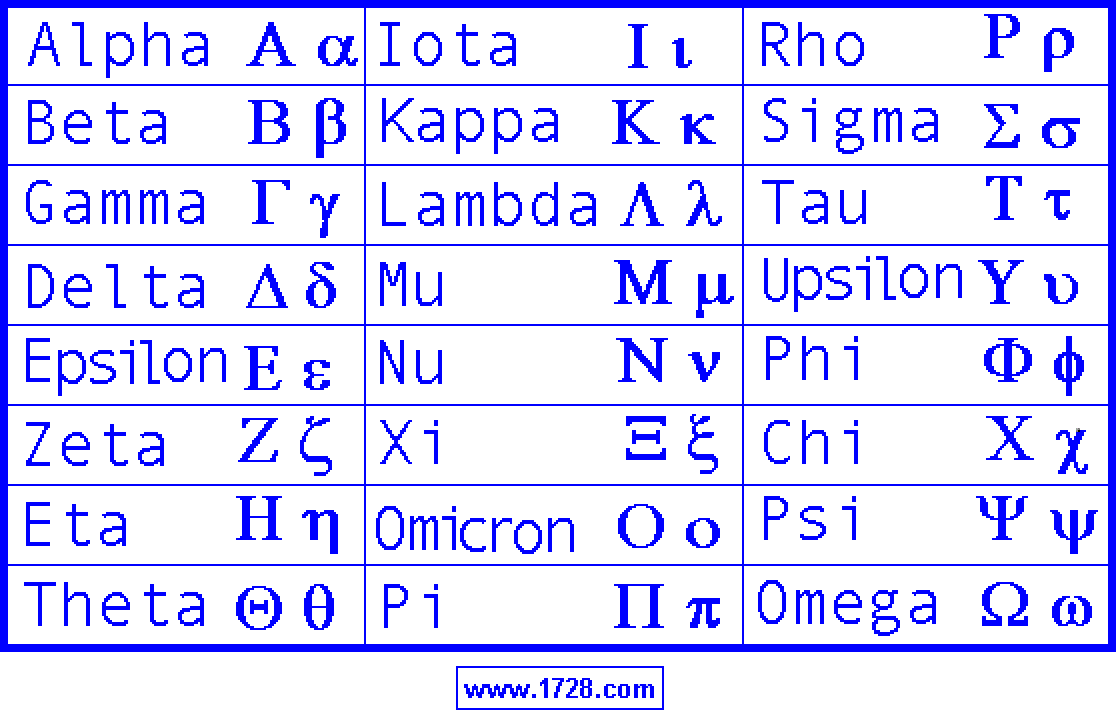The Greek Alphabet

 Alpha A   a Iota I   i Rho Ρ   ρ Beta B   b Kappa K   k Sigma Σ   σ Gamma G   g Lambda L   l Tau T   t Delta D   d Mu M   m Upsilon U   u Epsilon E   e Nu Ν   ν Phi Φ   φ Zeta Z   z Xi X   x Chi Χ   χ Eta Η   η Omicron O   o Psi Ψ   ψ Theta Θ   θ Pi P   p Omega Ω   ω(If you need to know the HTML codes for displaying letters of the Greek Alphabet please click here) The above 2 tables are the Greek Alphabet. It is a good idea to have some familiarity with the Greek alphabet because Greek letters are quite often used as abbreviations in science and mathematics.
 Generally speaking, the lower-case Greek letters are used more frequently than the capital letters. Here are some examples. Astronomy uses the lower case Greek letters to name the brighter stars in a constellation. The star Alpha Centauri is sometimes written as α Centauri. Physics uses the the Greek letter lambda λ to represent wavelength and the Greek capital letter omega Ω to represent electrical resistance in ohms.
 Mathematics makes extensive use of Greek letters. The most famous example is probably the number pi (3.14159265...) being represented as π. Another common use is for the Greek capital letter sigma Σ to represent a sum. If you have used Microsoft Excel™ you will note the Greek letter sigma as a toolbar icon to represent the sum function. See? Now you know what that "zig-zag" really means. These are just a few examples, but it gives you some idea of the frequent use of the Greek Alphabet in math and science.